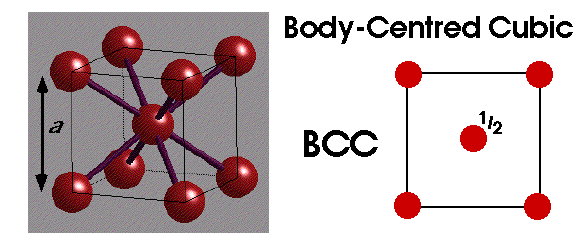# Problem: When spheres of radius r are packed into a body-centered cubic unit cell, they occupy 68.0% of the available volume. Use this to calculate the value of l, the length of the edge of the cell, in terms of r.A. l = 2.79r B. l = 1.44r C. l = 2.31r D. l = (4*21/2)r E. l = r

###### FREE Expert Solution

For this problem, we're being asked to determine the edge length of a body-centered cubic unit cell

Below is an image that shows the smallest unit cell that is repeatedly stacked for a BCC unit cellThe cell edge can be represented as "l" where the direction from a corner of cube to the farthest corner is the body diagonal "bd"

"fd" or face diagonal a line drawn from one vertex to the opposite corner of the same face. If the edge is "l", we can write an equation as:

Atoms along the body diagonal touch each other which makes the length of bd as 4 times the radius "r"

100% (260 ratings)###### Problem Details

When spheres of radius r are packed into a body-centered cubic unit cell, they occupy 68.0% of the available volume. Use this to calculate the value of l, the length of the edge of the cell, in terms of r.

A. l = 2.79r

B. l = 1.44r

C. l = 2.31r

D. l = (4*21/2)r

E. l = r